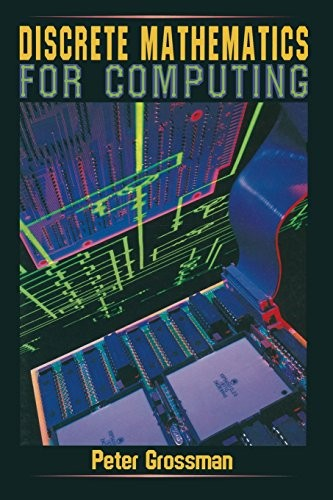# Discrete Mathematics for Computing by Peter Grossman

In Stock
£23.99
This introduction to discrete mathematics, aimed at students with a limited background in mathematics, presents the material that forms the essential background for studies in computing and information systems.
Only 1 left

## Discrete Mathematics for Computing Summary

### Discrete Mathematics for Computing by Peter Grossman

Written in a clear style and aimed at students with a limited background in mathematics, this introduction to discrete mathematics presents the material that forms the essential background for studies in computing and information systems. The topics covered include number systems, logic, relations, functions, induction, recursion, Boolean algebra, combinatorics, graph theory and number theory. The text contains many examples of applications of the theory to problems in computing, including a chapter on the representation and manipulation of numbers in a computer, examples of the use of propositional logic in program design, a section discussing the relationship between the mathematical and programming concepts of a function, and a chapter on the estimation of the time complexity of algorithms. A feature of the book is the use of algorithms written in pseudo code in many examples throughout the text. The exercises at the end of each chapter provide both routine drill of basic techniques and more challenging problems designed to enhance and extend the student's understanding. Answers to most of the exercises are provided at the back of the book. This book should be suitable for use as a text for a one-semester introductory course in discrete mathematics, particularly for students who are undertaking studies in computing and information systems.

### Why buy from World of BooksOur excellent value books literally don't cost the earthFree delivery in the UKEvery used book bought is one saved from landfill

PETER GROSSMAN has worked in both academic and industrial roles as a mathematician and computing professional. As a lecturer in mathematics, he was responsible for coordinating anddeveloping of mathematics courses for Computer Science students.He is based in Australia and currently works in industry, in the areas of mathematical modelling and software development."

Introduction to Algorithms - Bases and Number Representation - Computer Representation and Arithmetic - Logic - Sets and Relations - Functions - Induction and Recursion - Boolean Algebra and Digital Circuits - Combinatorics - Introduction to Graph Theory - Trees - Number Theory - Algorithms and Computational Complexity - Answers to Exercises - Index

GOR001137171
Discrete Mathematics for Computing by Peter Grossman
Peter Grossman
Used - Very Good
Paperback
Palgrave Macmillan
1995-03-15
304
0333646940
9780333646946
N/A
Book picture is for illustrative purposes only, actual binding, cover or edition may vary.
This is a used book - there is no escaping the fact it has been read by someone else and it will show signs of wear and previous use. Overall we expect it to be in very good condition, but if you are not entirely satisfied please get in touch with us.